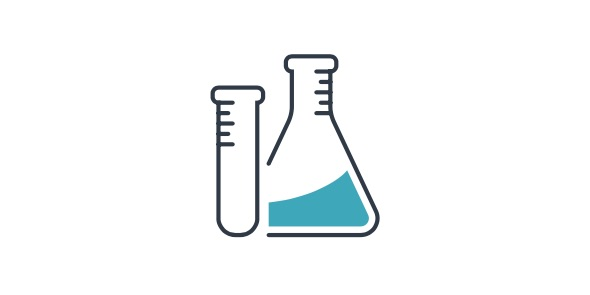# 701stilwell - 7th Grade - Science - Benchmark 1

26 Questions | Total Attempts: 441SettingsIn order for one to embark on an experiment they must come up with a hypothesis to which they base their experiment on. If there is no hypothesis there is no experiment. How much do you know about benchmarking when it comes to experiments? Give the quiz a try and find out.

• 1.
1. A testable prediction about a possible solution to a problem is called____________.
• A.

a conclusion

• B.

an experiment

• C.

A hypothesis

• D.

A variable

• 2.
2. When designing an experiment, the first step is to_____________.
• A.

Analyzing the data

• B.

List a procedure

• C.

State a hypothesis

• D.

State the problem

• 3.
3. A factor that does NOT change in an experiment is a(n)_____________.
• A.

Constant

• B.

Control

• C.

Dependent variable

• D.

Independent variable

• 4.
4. People do experiments to test the effects of_____________.
• A.

Conclusions

• B.

Hypotheses

• C.

Independent variables

• D.

Dependent variables

• 5.
5. Factors that are measured in an experiment are the_______________.
• A.

Hypotheses

• B.

Independent variables

• C.

Dependent variables

• D.

Constants

• 6.
6. Step-by-step procedures of scientific problem solving are called_____________.
• A.

Scientific methods

• B.

Hypotheses

• C.

Conclusions

• D.

Inferences

• 7.
7. The temperature of a liquid, the height of a plant, or an observation that one object is smaller than another are all examples of___________________.
• A.

Data

• B.

Hypotheses

• C.

Inferences

• D.

Experiments

• 8.
8. A judgment based on what has been observed in an experiment is a(n)______________.
• A.

Hypothesis

• B.

Observation

• C.

Dependent variable

• D.

Conclusion

• 9.
9. An explanation of an observation is a(n)_______________.
• A.

Experiment

• B.

Independent variable

• C.

Inference

• D.

Dependent variable

• 10.
10. After scientists reach conclusions from the results of their experiments, they ______________.
• A.

Do nothing

• B.

Communicate their results to other people

• C.

Form a hypothesis

• D.

Analyze their data

• 11.
11. In an experiment testing the amount of sunlight that is best for growing plants, the height of the plant is the____________________.
• A.

Independent variable

• B.

Hypothesis

• C.

Conclusion

• D.

Dependent variable

• 12.
12. The measurement of an object's mass is a ___________________.
• A.

Physical change

• B.

Physical property

• C.

Chemical change

• D.

Chemical property

• 13.
13. The ability of an apple to change color when exposed to air is a ________________.
• A.

Physical property

• B.

Chemical property

• C.

Physical change

• D.

Chemical change

• 14.
14. The ability of a pond to freeze over in winter is a ______________.
• A.

Physical property

• B.

Chemical property

• C.

Physical change

• D.

Chemical change

• 15.
15. When a newspaper is left in direct sunlight for a few days, the paper begins to turn yellow. The yellow color is evidence of a __________________.
• A.

Physical property

• B.

Chemical property

• C.

Physical change

• D.

Chemical change

• 16.
16. When a chunk of dry ice is at room temperature, a cloud of carbon dioxide vapor forms. The carbon dioxide is changing from a _____________________.
• A.

Solid to a liquid

• B.

Solid to a gas

• C.

Liquid to plasma

• D.

Liquid to a gas

• 17.
17. Three examples of physical change are ____________________.
• A.

Boiling water, a nail rusting, a melting candle

• B.

A pond freezing, breaking glass, a burning candle

• C.

Melting ice, mowing the lawn, carving a statue

• D.

• 18.
Match the statement with the correct term.18. The building is red.
• A.

Observation

• B.

Conclusion

• 19.
Match the statement with the correct term.19. The water is hot.
• A.

Observation

• B.

Conclusion

• 20.
Match the statement with the correct term.20. Plants will die if they are watered to little or to much.
• A.

Observation

• B.

Conclusion

• 21.
Match the statement with the correct term.21. It is going to rain.
• A.

Observation

• B.

Conclusion

• 22.
Match the statement with the correct term.22.The liquid smells like peppermint.
• A.

Observation

• B.

Conclusion

• 23.
Match the example of chemical change with the correct sign of chemical change.23. burning wood in a fireplace
• A.

The formation of a precipitate

• B.

A change in color

• C.

Release of light and heat

• D.

The formation of a gas

• 24.
Match the example of chemical change with the correct sign of chemical change.24. antacid tablet in water
• A.

The formation of a precipitate

• B.

A change in color

• C.

Release of light and heat

• D.

The formation of a gas

• 25.
Match the example of chemical change with the correct sign of chemical change.25. solution of sodium nitrate is mixed with a solution of lead nitrate
• A.

The formation of a precipitate

• B.

A change in color

• C.

Release of light and heat

• D.

The formation of a gas

Related TopicsBack to top